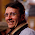## Monday, August 23, 2010

### A lillte puzzle with balls

Little interview puzzle: A container is filled up with balls. Each ball has a value. Red = 7, Yellow = 5, Green = 2, Blue 2. Some balls are removed from the container and the product of their value is 147,000. How many red balls have been removed?

#### 6 comments:

1.did you mean green=3?

2.Shouldn't one color have value 3?

3.4.5.suppose we remove a red balls, b yellow, c green and d blue balls.

Then 7^a * 5^b * 3^c * 2^d = 147000

The following piece of code finds that a=2,
b=3, c=1, d=3. Hence, we removed 2 red balls.

int main() {
int a = 0, b = 0, c = 0, d = 0;
int product = 0;
for (a = 0; a < 10; a++) {
for (b = 0; b < 10; b++) {
for (c = 0; c < 10; c++) {
for (d = 0; d < 10; d++) {
product = pow(7,a) * pow(5,b) * pow(3,c) * pow(2,d);
if (product == 147000) {
printf("Found Values: a=%d, b=%d, c=%d, d=%d.\n", a, b, c, d);
}
}
}
}
}
}

6.2 red balls.

147000 = 2x2x2x3x5x5x5x7x7

PS of course the value of the green or the blue color must be 3.